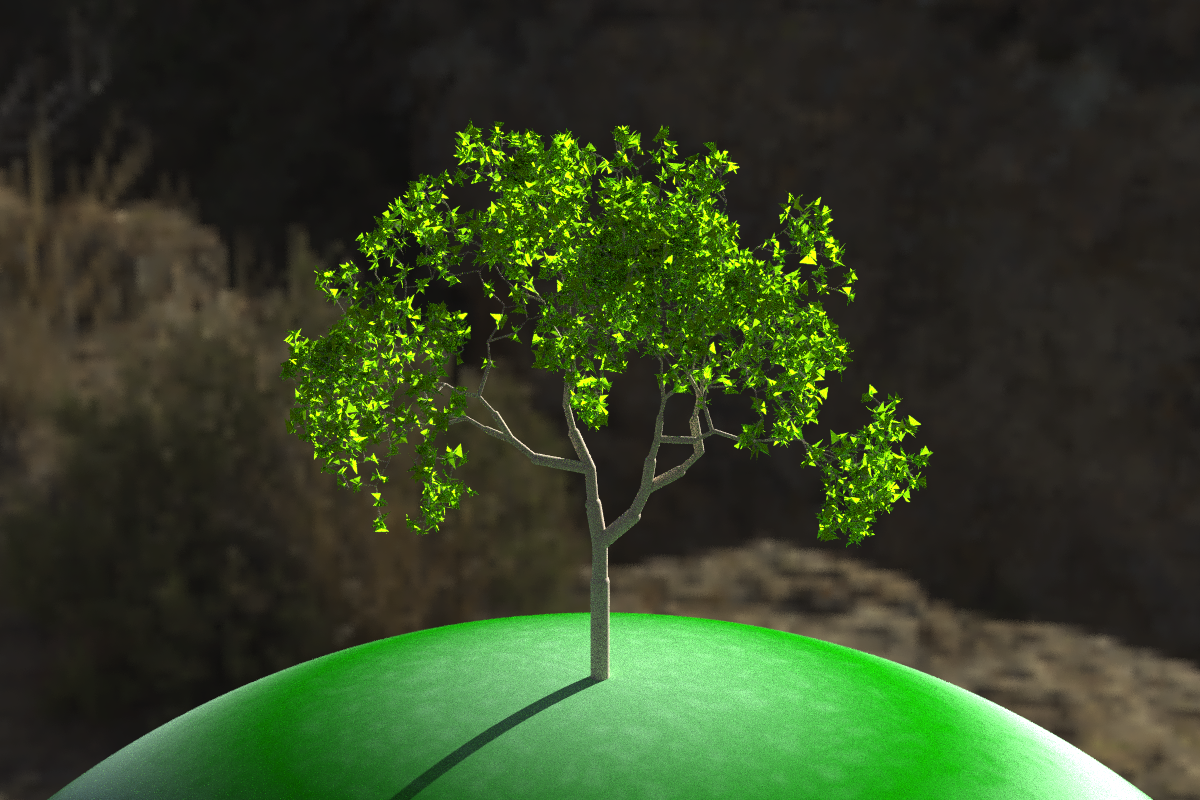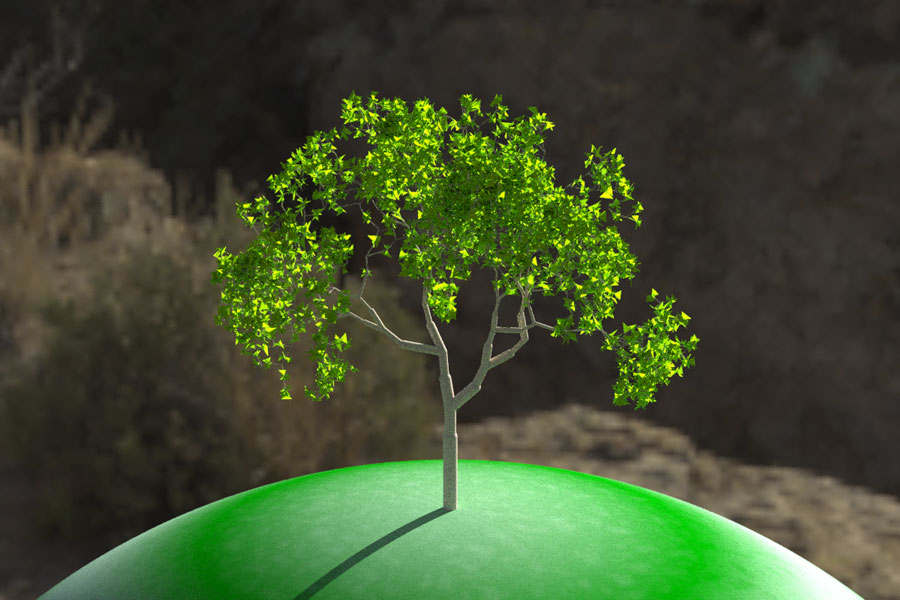raybonsai: Generate 3D procedural trees in R, rendered with rayrender! Procedural generation code based on the flametree package by Danielle Navarro.

## Installation

You can install raybonsai with the remotes package:

remotes::install_github("tylermorganwall/raybonsai")

## Example

There are currently only two user-facing functions in raybonsai: generate_tree() and render_tree(). generate_tree() generates a tree that follows a certain set of constraints that you set and returns a rayrender scene describing the tree. render_tree() automatically adds ground, sets up lighting, and sets up the camera so the tree is in frame, but is otherwise just a light wrapper around rayrender’s render_scene() function.

Here are some examples:

library(raybonsai)
library(rayrender)

generate_tree() %>%
render_tree(width=800, height=800)Change the branch angles and depth of the tree:

generate_tree(seed = 4321, branch_angle_vert = seq(-10,20,by=1),
branch_depth = 8, leaf_color = "yellow", leaf_depth_start = 5) %>%
render_tree(width=800, height=800)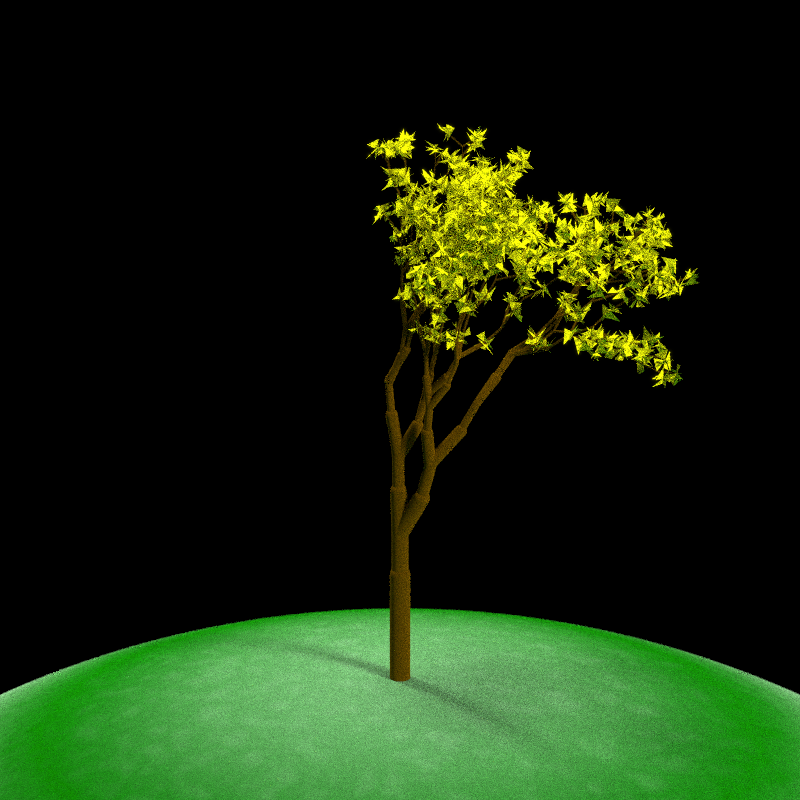Adjust the colors of the ground and the tree:

generate_tree(seed = 20, branch_angle = c(-30,0, 30), branch_scale = c(0.9,1),
branch_depth = 7, leaf_color = "chartreuse4", leaf_depth_start = 5, branch_color = "tan") %>%
render_tree(ground_color1 = "darkgoldenrod4", ground_color2 = "chocolate4",
width=800, height=800)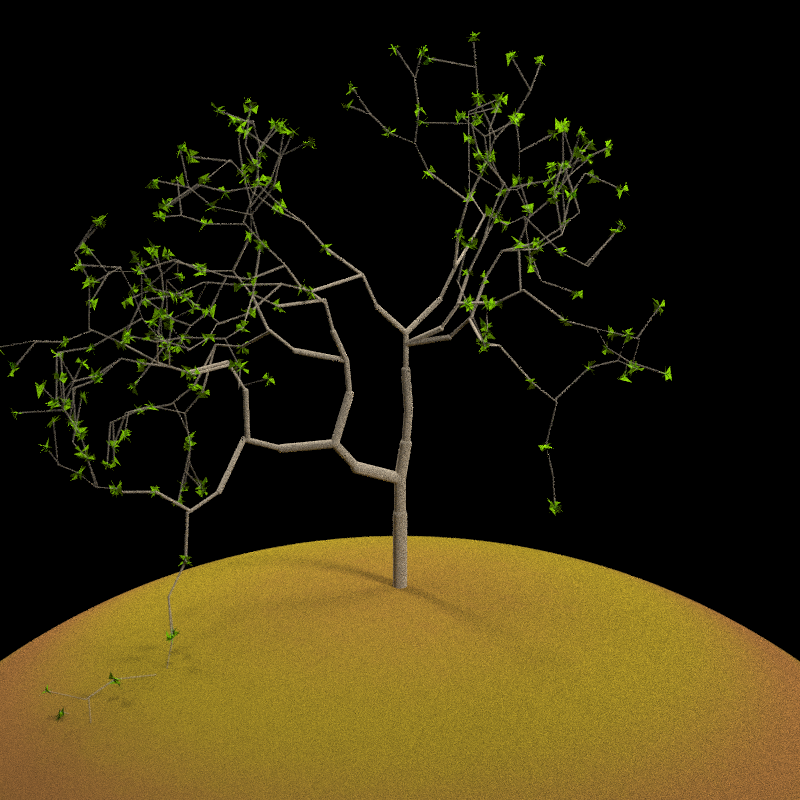generate_tree(seed = 222, branch_angle = c(-20, 20), branch_scale = c(0.8,0.9), branch_split = 3,
branch_depth = 6 , leaf_color = "chartreuse4", leaf_depth_start = 5, branch_color = "tan") %>%
render_tree(lights = FALSE, ground_color1 = "grey50",ground_color2 = "grey50", width=1200,height=800)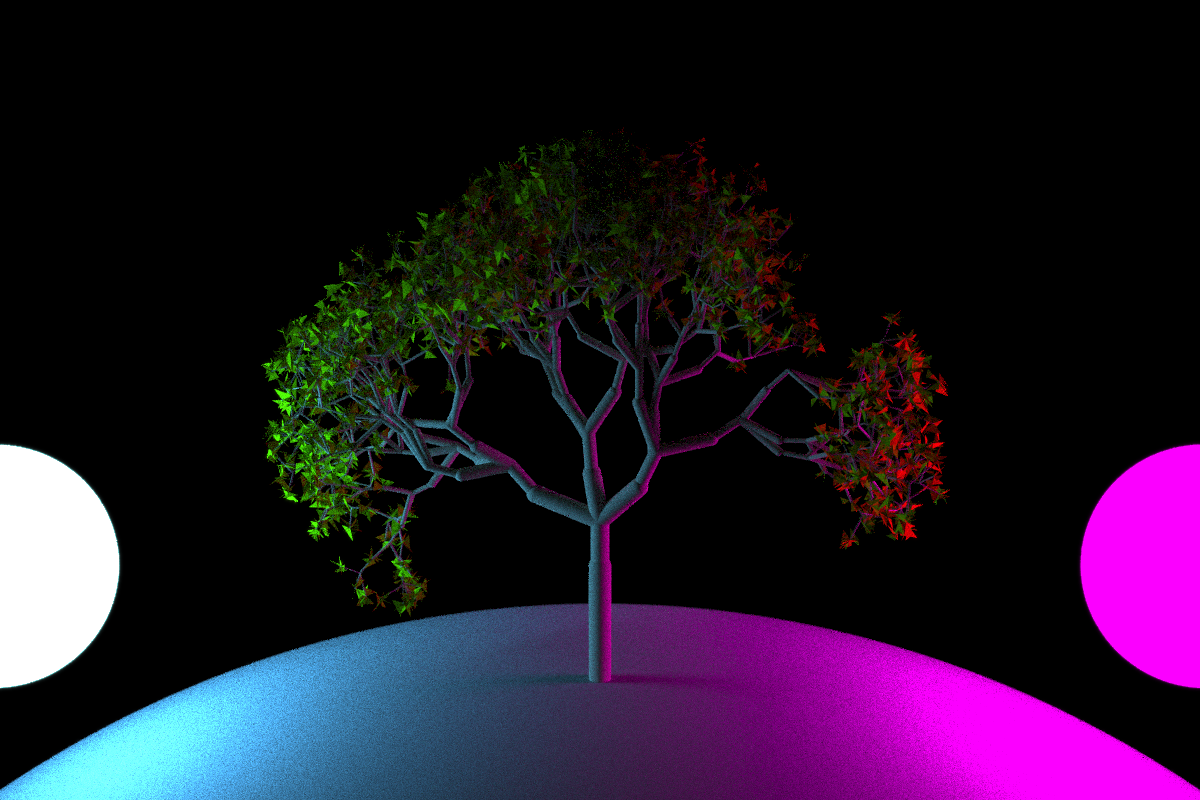generate_tree(seed = 222, branch_angle = c(-20,20), branch_scale = c(0.8,0.9),
render_tree(lights = FALSE, environment_light = "~/Desktop/hdr/kiara_3_morning_2k.hdr", width=1200, height=800)